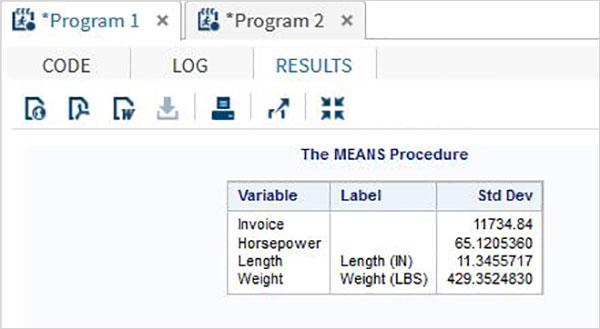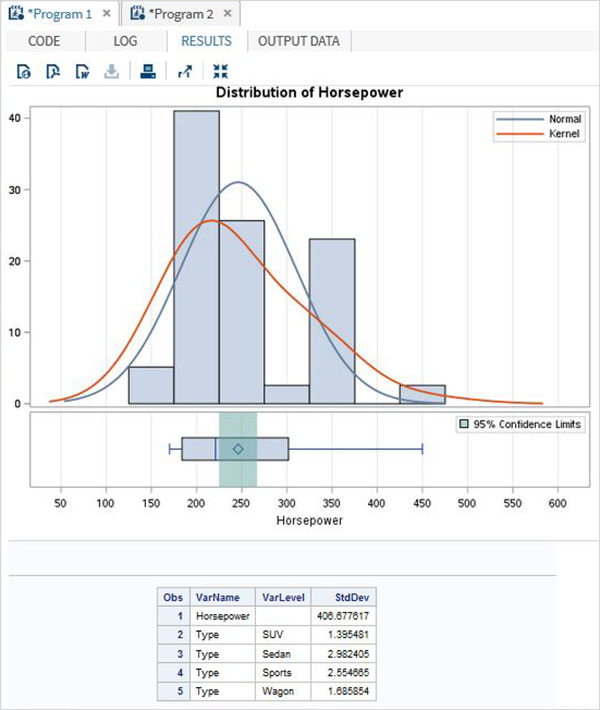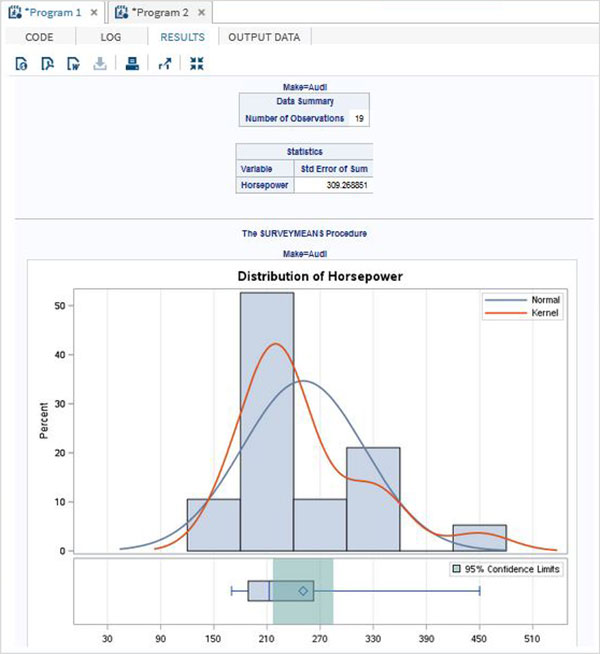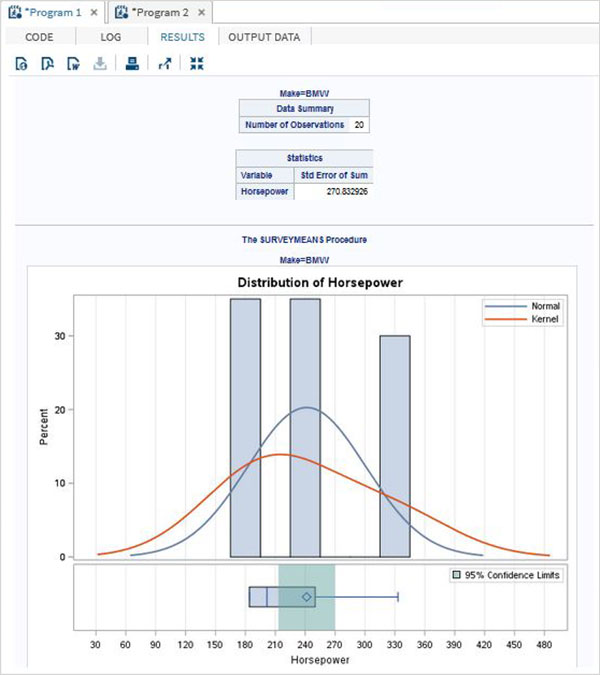# SAS - Standard Deviation

Standard deviation (SD) is a measure of how varied is the data in a data set. Mathematically it measures how distant or close are each value to the mean value of a data set. A standard deviation value close to 0 indicates that the data points tend to be very close to the mean of the data set and a high standard deviation indicates that the data points are spread out over a wider range of values

In SAS the SD values is measured using PROC MEAN as well as PROC SURVEYMEANS.

## Using PROC MEANS

To measure the SD using proc means we choose the STD option in the PROC step. It brings out the SD values for each numeric variable present in the data set.

### Syntax

The basic syntax for calculating standard deviation in SAS is −

```PROC means DATA = dataset STD;
```

Following is the description of the parameters used −

• Dataset − is the name of the dataset.

### Example

In the below example we create the data set CARS1 form the CARS data set in the SASHELP library. We choose the STD option with the PROC means step.

```PROC SQL;
create table CARS1 as
SELECT make, type, invoice, horsepower, length, weight
FROM
SASHELP.CARS
WHERE make in ('Audi','BMW')
;
RUN;

proc means data = CARS1 STD;
run;
```

When we execute the above code it gives the following output −## Using PROC SURVEYMEANS

This procedure is also used for measurement of SD along with some advance features like measuring SD for categorical variables as well as provide estimates in variance.

### Syntax

The syntax for using PROC SURVEYMEANS is −

```PROC SURVEYMEANS options statistic-keywords ;
BY variables ;
CLASS variables ;
VAR variables ;
```

Following is the description of the parameters used −

• BY − indicates the variables used to create groups of observations.

• CLASS − indicates the variables used for categorical variables.

• VAR − indicates the variables for which SD will be calculated.

### Example

The below example describes the use of class option which creates the statistics for each of the values in the class variable.

```proc surveymeans data = CARS1 STD;
class type;
var type horsepower;
ods output statistics = rectangle;
run;
proc print data = rectangle;
run;
```

When we execute the above code it gives the following output −## Using BY option

The below code gives example of BY option. In it the result is grouped for each value in the BY option.

### Example

```proc surveymeans data = CARS1 STD;
var horsepower;
BY make;
ods output statistics = rectangle;
run;
proc print data = rectangle;
run;
```

When we execute the above code it gives the following output −

### Result for make = "Audi"### Result for make = "BMW"